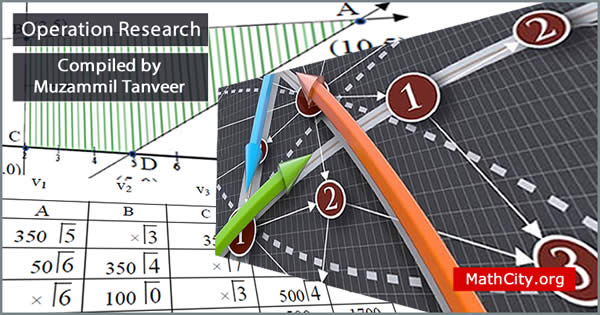# Operation Research by Sir Haidar AliThese notes are provided and composed by Mr. Muzammil Tanveer. We are really very thankful to him for providing these notes and appreciates his effort to publish these notes on MathCity.org. These are based on lectures delivered by Sir Haidar Ali at GC University Faisalabad.

 Name Operation Research Handwritten Notes Sir Haidar Ali Muzammil Tanveer 70 pages PDF 916 kB
• Linear programming
• Linear function
• Objective function
• Optimal solution
• General linear programming problem
• Graphical solution of linear programming problem
• Corner point
• Infeasible region
• Unbounded solution
• Simplex method for solving linear programming problem
• Surplus variable
• Initial basic feasible solution
• Gauss jordan method
• Special case in simplex method
• Degeneracy
• Unrestricted variable
• Two phase method
• Big-m method or m-techniqu
• Revised simplex method or dual simplex method
• Duality in linear programming
• Transportation problem
• Special case degenerate / degeneracy
• Least cost method
• Vogel’s approximation method or method of penalty
• Optimal solution for transportation problem
• Modified distribution method or modi method or u-v method
• Maximization in transportation problem
• Assignment problem
• Unbalance problem in assignment problem
• Network minimization
• Graph/network
• Connected graph

Please click on View Online to see inside the PDF.

• msc/notes/operation-research-haidar-ali